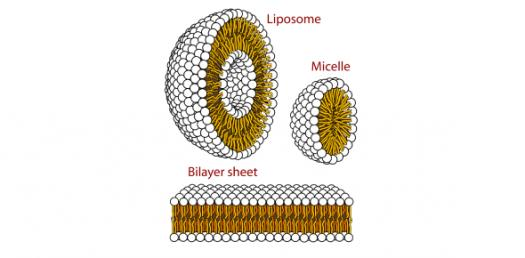41 Questions | Attempts: 109
ShareSettingsThe quiz below is designed to Test Your Knowledge about Membrane Biology! The membrane is the outer covering of a cell and it is in charge of bringing material in and out of the cell and protects the cell from the outer environment. Do take up the quiz and get to see what more you will get to learn about the cell membrane.

• 1.
What is the current model of cell membranes?
• A.

Extracellular model

• B.

Phospholipid model

• C.

Cellular model

• D.

Fluid-mosaic model

• 2.
What does the fluid part of the fluid-mosaic model mean?
• A.

Phospholipids move laterally (side to side)

• B.

Phospholipids move longitudinally (up and down)

• C.

Proteins are embedded in the phospholipid bilayer

• D.

Carbohydrates are embedded in the phospholipid bilayer

• 3.
What does the mosaic part of the fluid-mosaic model mean?
• A.

Phospholipids move laterally (side to side)

• B.

Phospholipids move longitudinally (up and down)

• C.

Proteins are embedded in the phospholipid bilayer

• D.

Carbohydrates are embedded in the phospholipid bilayer

• 4.
What can increase fluidity in membranes?
• A.

Cholesterol

• B.

Unsaturated fatty acids

• C.

Saturated fatty acids

• D.

Glucose

• E.

A and B

• F.

A and C

• 5.
What are the functions of an embedded protein?
• A.

Transport of polar substances across membrane

• B.

Enzyme activity/sequencing

• C.

Signal transduction/cell communication

• D.

Cell to cell regulation (CCM)

• E.

Intercellular junctions (tight and gap)

• F.

To attach ECM to cell

• G.

A and C

• H.

D and E

• I.

All of the above

• J.

None of the above

• 6.
What does it mean to be selectively permeable?
• A.

Let anything cross

• B.

Let only certain stuff cross

• C.

Let nothing cross

• D.

None of the above

• 7.
How do non-polar substances cross membrane?
• A.

Easily, go straight through bilayer

• B.

Crosses at the next level

• C.

By going through the protein

• D.

None of the above

• 8.
How do polar substances cross membrane?
• A.

Easily, go straight through bilayer

• B.

Crosses at the next level

• C.

By going through the protein

• D.

None of the above

• 9.
How do ionic substances cross membrane?
• A.

Easily, go straight through bilayer

• B.

Crosses at the next level

• C.

By going through the protein

• D.

None of the above

• 10.
What facilitates the movement of water in a membrane?
• A.

Lipids called aquaporins

• B.

Proteins called aquaporins

• C.

Lipids called hydroglycins

• D.

Proteins called hydroglycins

• 11.
What is diffusion?
• A.

Low to high concentration, passive transport

• B.

Low to high concentration, active transport

• C.

High to low concentration, passive transport

• D.

High to love concentration, active transport

• 12.
What is osmosis?
• A.

Active transport of water

• B.

Diffusion of water

• C.

Facilitate diffusion of a protein

• D.

None of the above

• 13.
What is the hypotonic part of the solution?
• A.

Most water, least other thing

• B.

Least water, most other thing

• C.

Equal water and other thing

• D.

None of the above

• 14.
What is the hypertonic part of the solution?
• A.

Most water, least other thing

• B.

Least water, most other thing

• C.

Equal water and other thing

• D.

None of the above

• 15.
What does it mean if a solution is isotonic?
• A.

Most water, least other thing

• B.

Least water, most other thing

• C.

Equal water and other thing

• D.

None of the above

• 16.
What is the formula for water potential?
• A.

Psi = Psi W + Psi S

• B.

Psi S = -iCRT

• C.

Psi = Psi P + Psi S

• D.

Psi = -iCRT

• 17.
What does Psi stand for?
• A.

Water potential

• B.

Pressure potential (usually zero)

• C.

Solute potential

• D.

Molar concentration

• 18.
What does Psi P stand for?
• A.

Water potential

• B.

Pressure potential (usually zero)

• C.

Solute potential

• D.

Molar concentration

• 19.
What does Psi S stand for?
• A.

Water potential

• B.

Pressure potential (usually zero)

• C.

Solute potential

• D.

Molar concentration

• 20.
How do you find Psi S?
• A.

-CRT

• B.

CRT

• C.

-iCRT

• D.

ICRT

• 21.
In Psi S = -iCRT, what is i?
• A.

Ionication constant

• B.

Molar concentration

• C.

Gas Constant (0.0831)

• D.

Temperature in Kelvin

• 22.
In Psi S = -iCRT, what is C?
• A.

Temperature in Kelvin

• B.

Ionication constant

• C.

Gas Constant (0.0831)

• D.

Molar concentration

• 23.
In Psi S = -iCRT, what is R?
• A.

Molar concentration

• B.

Gas constant (0.0831)

• C.

Temperature in Kelvin

• D.

Ionication constant

• 24.
In Psi S = -iCRT, what is T?
• A.

Temperature in Kelvin

• B.

Molar concentration

• C.

Ionication constant

• D.

Gas constant (0.0831)

• 25.
How do you get Kelvin?
• A.

Fahrenheit - 273

• B.

Celcius - 273

• C.

Fahrenheit + 273

• D.

Celcius + 273Back to top
×

Wait!
Here's an interesting quiz for you.